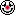View Single Post
 peter_budoAuthorized User Join Date: Aug 2004 Location: Jasenie, , Slovakia. Posts: 14 Thanks: 0 Thanked 0 Times in 0 PostsReading from external data file

Hi
I'm looking for somebody who can help me to understand how to read from external file without "destroing" existing inforamtion inside.

whole staff is based on book example which i'm trying to extend.
I have a programm which is working with database of 3 three accounts.
There is no problem to do operation on it(Deposit, Withdraw) and update data file. However if I insert there on start extra line which state how many accounts are there everything gets funywell i tried to use atoi to change string variable to int but doesnt like it, it's saying
49: cannot convert `na' from type `string' to type `const char *'
I tried create another variable type integer and than convert string but than there message
cannot convert `na' from type `string' to type `const char *'

Anything else I can do with it???
Here is code as is look

data file
3 accounts

123 100.5
840 123.43
666 8273.06

//account11.cc based on notes from tutorial Peter
#include<iostream.h>
#include<fstream.h>
#include<string>

const int ACC_MAX = 10;
enum TransactionType {Deposit, Withdraw};

struct Account //grouping account number and balance under Account
{
int accno;
double balance;
};
struct Transaction //grouping transaction type, account number and amount of money moved{
TransactionType type;
int accno;
double amount;
};

void process(Transaction t, Account& a) //if deposit add money, if withdraw take out money
{
switch(t.type)
{
case Deposit:
a.balance += t.amount;
break;
case Withdraw:
a.balance -= t.amount;
break;
}
}

void main()
{
Account accs[ACC_MAX];

//open the data file
ifstream accfile;
accfile.open("accounts.dat");

string na; //number of accounts
accfile >> accs.accno;
getline (accfile, ucty);
atoi(na);
cout <<"Reading from first line = " << na << endl;

do //this does counting of accounts but I want to replace with stuff above
{ //where reading from first line says how many accounts are there
if(accfile)
{
}
} while (accfile && nRead < ACC_MAX);

Transaction trans = {Withdraw, 123, 50.0}; //simple transaction WITHDRAW from account 123
int a=0;
for (int i =0; i < nRead; i++)
{
if (accs[i].accno == trans.accno)
{
process (trans, accs[i]);
a = i;
}
}

//check the balance //print out up-date of account
cout <<"Account number: " <<accs[a].accno << endl;
cout <<"Balance is: £" << accs[a].balance << endl;
accfile.close();

ofstream oaccfile; //save any changes made
oaccfile.open("accounts.dat");
for (int i = 0; i < nRead; i++)
{
oaccfile << accs[i].accno << " " << accs[i].balance << endl;
}

oaccfile.close();
}

P.T.
__________________
P.T.